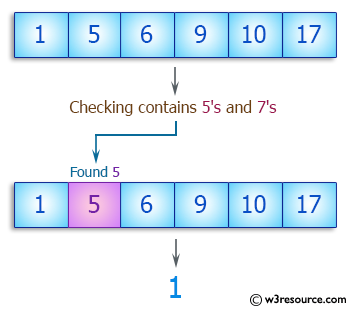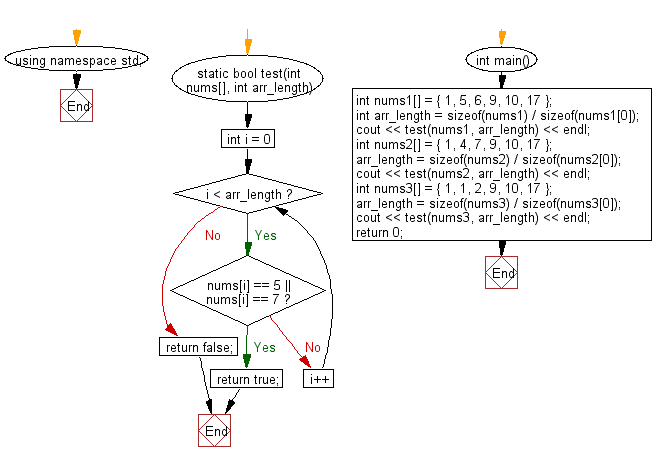﻿ C++ Exercises: Check whether a given array of integers contains 5's and 7's - w3resource# C++ Exercises: Check whether a given array of integers contains 5's and 7's

## C++ Basic Algorithm: Exercise-103 with Solution

Write a C++ program to check whether a given array of integers contains 5's and 7's.

Sample Solution:

C++ Code :

``````#include <iostream>

using namespace std;

static bool test(int nums[], int arr_length)
{
for (int i = 0; i < arr_length; i++)
{
if (nums[i] == 5 || nums[i] == 7) return true;
}

return false;
}

int main()
{
int nums1[] = { 1, 5, 6, 9, 10, 17 };
int arr_length = sizeof(nums1) / sizeof(nums1);
cout << test(nums1, arr_length) << endl;
int nums2[] = { 1, 4, 7, 9, 10, 17 };
arr_length = sizeof(nums2) / sizeof(nums2);
cout << test(nums2, arr_length) << endl;
int nums3[] = { 1, 1, 2, 9, 10, 17 };
arr_length = sizeof(nums3) / sizeof(nums3);
cout << test(nums3, arr_length) << endl;
return 0;
}
``````

Sample Output:

```1
1
0
```

Pictorial Presentation:Flowchart:C++ Code Editor:

Contribute your code and comments through Disqus.

What is the difficulty level of this exercise?

﻿